# GMAT Math : DSQ: Calculating the diagonal of a prism

## Example Questions

### Example Question #7 : Prisms

Find the length of the diagonal of cube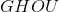.

I)has a volume of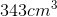.

II)has a surface area of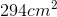.

Either statement is sufficient to answer the question.

Both statements are needed to answer the question.

Statement II is sufficient to answer the question, but statement I is not sufficient to answer the question.

Statement I is sufficient to answer the question, but statement II is not sufficient to answer the question.

Either statement is sufficient to answer the question.

Explanation:

To find the length of the diagonal, we need the side length.

I) Gives us the volume of the cube. Take the cubed root to find the side length.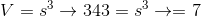II) Gives us the surface area, divide by 6 (the number of faces in a cube) and take the square root to find the side length.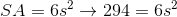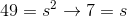Diagonal of a cube is the side length times the square root of three. Alternatively, this can be found using the Pythagorean Theorem twice.

Either way, we can use I) or II) to find our side lenght and then our diagonal.

### Example Question #8 : Prisms

What is the diagonal of a rectangular prism?

1) Its surface area is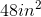2) Its height = twice its width = thrice its length.

Together, the two statements are sufficient.

Either of the statements is sufficient.

Neither of the statements, separate or together, is sufficient.

Statement 1 alone is sufficient.

Statement 2 alone is sufficient.

Together, the two statements are sufficient.

Explanation:

The diagonal of a rectangular prism is found via the formulaThe second statement reduces this to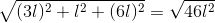However, the actual length is unknown. Statement 1 allows the calculation of a numerical value: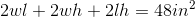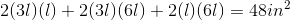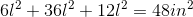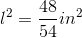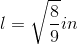Tired of practice problems?

Try live online GMAT prep today.